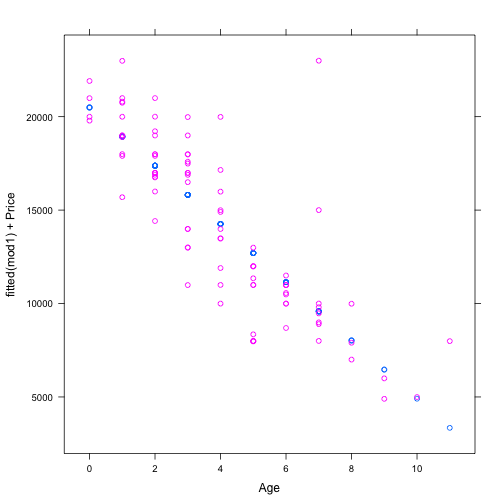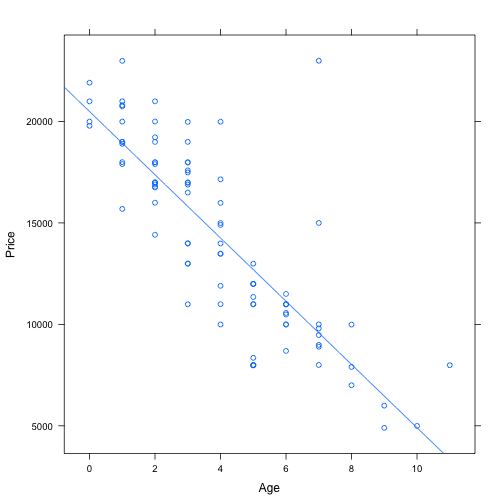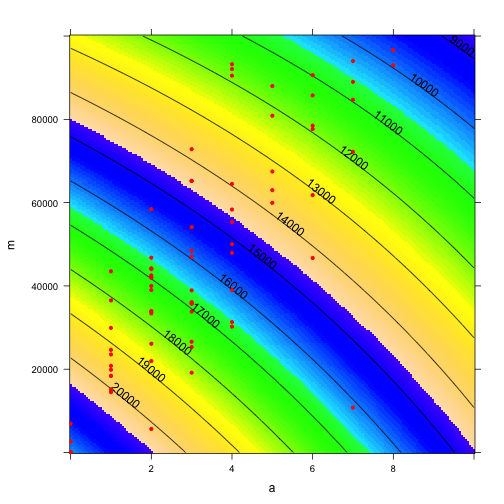# Survey Project

1. Show the project description on the Moodle site.
3. Have students form groups around common interests. Enter their information on the Google Doc.

# Review

$$R^2$$ between zero and 1.

• Use delta with nested models to decide if a model is better than junk.
• SAT versus GPA, $$R^2 \approx 0.16$$. Based on thousands of student records.
• Little $$r$$ and the meaning of $$r=0.40$$

fetchData("M155/littleR.R")

## Retrieving from http://www.mosaic-web.org/go/datasets/M155/littleR.R

##  TRUE


Run littleR.

The blue vector is constructed from a linear combination of a red vector and a black vector. Move the slider to change the amount of the black vector that goes into the sum.

The vectors are being displayed in both variable space and case space. Notice how the roundness of the case-space cloud reflects the angle. The correlation coefficient, r, corresponds to the roundness and to the angle $$\theta$$ between the blue and black vectors.

### Strategies for Including Explanatory Terms

• Silly: Just ignore everything. Why is this silly?
• Naive: Just throw in everything. The disadvantages of this are more subtle to understand but can be devastating.
• Monitor R2. Only include things that give a substantial increase in R2. Later in the semester, this will lead to our consideration of the strength of evidence.
• Isolating effects/controlling for/adjusting for: What covariates to hold constant?
• Mechanistic: Looking at causal pathways. We'll get to this at the end of the semester.

• What does a GPA look like from a modeling perspective?
• What other variables might influence grades?
• Which of these do we want to control for?

Suppose that a new measure of academic achievement were proposed. How would you decide if it's better than GPA?

# Partial versus total

Suppose I have a used car. I'm going away for a year and thinking of selling it. On the other hand, it would be nice to have a car available when I get back. How much will it cost me to delay selling the car for a year?

Consider several models of used car prices fitted to data on used Hondas:

cars = fetchData("used-hondas.csv")

## Retrieving from http://www.mosaic-web.org/go/datasets/used-hondas.csv

mod1 = lm(Price ~ Age, data = cars)
mod2 = lm(Price ~ Mileage, data = cars)
mod3 = lm(Price ~ Mileage + Age, data = cars)
mod4 = lm(Price ~ Mileage * Age, data = cars)


Each of the first three is nested in the 4th. You can play around with the various models this way:

fetchData("mLM.R")
mLM(Price ~ Age * Mileage, data = cars)


Include and exclude terms to try to answer this question:

Which is the right model to use to inform my car-selling decision?

Tempting to use model 1, since Age is the only variable that I'm interested in.

xyplot(fitted(mod1) + Price ~ Age, data = cars)It's a bit hard to see the model. Let's try another way of plotting it.

f1 = makeFun(mod1)
plotPoints(Price ~ Age, data = cars)
plotFun(f1(Age) ~ Age, add = TRUE)How much the price goes down with a year depends on how old the car is, but you can get the rate from the derivative of the function. Let's evaluate that derivative for an 8-year old car with 50,000 miles:

f1 = makeFun(mod1)
df1 = D(f1(Age) ~ Age)
df1(Age = 8)

##     1
## -1559


Or, since I'm really thinking about a 1-year difference:

f1(Age = c(9, 8), Mileage = 50000)

##    1    2
## 6465 8023


Take the difference. QUESTION: How come I get the same answer for the finite-difference and the derivative?

But let's consider mod4

f4 = makeFun(mod4)
plotFun(f4(Age = a, Mileage = m) ~ a & m, a.lim = c(0, 10), m.lim = c(0, 1e+05),
levels = 1000 * (1:20), npts = 200)
plotPoints(Mileage ~ Age, data = cars, add = TRUE, pch = 20, col = "red")Examine the change as age goes up by one year. Should I hold mileage constant or should I let the mileage change with age in the typical way?

Here's the same question another way: Do I want to compare cars with different mileages and different ages, or do I want to compare cares with different ages and the same mileage.

Calculating the partial derivative or partial change:

• Take a finite-difference approach
f4(Age = c(8, 9), Mileage = 50000)

##     1     2
## 12815 12238

• Use partial derivatives
df4da = D(f4(Age = Age, Mileage = Mileage) ~ Age)
df4da(Age = 8, Mileage = 50000)

##    1
## -577

• Do it analytically, using the coefficients to construct the model polynomial.

### Review of Partial Derivatives

Relate to the two-variable polynomial: $$f(x,y) = a_0 + a_1 x + a_2 y + a3 x y + ...$$

Relate to the three-variable polynomial.

• Calculation from model function by evaluating at nearby points

### Real-world examples

• Insurance example: Should we pay people not to be on the College's health plan?
• NGOs and development aid: Speaker Christie Hansen talked about how donors for health funding in Kenya ended up funding other activities as the government moved money away from health toward agriculture, etc.
• Merit aid. Will it reduce net tuition or increase it. Depends on whether we substantially change the yield.

### Activity

Total-vs-partial In-class activity

### Discussion

#### Kids feet data.

The question is whether girls' shoes are narrower than boys' because girls' feet are narrower. Address this.

#### Wages

• Is there discrimination on the basis of sex? Build a potentially complicated model and look at the partial change with respect to sex?
• What's the effect of education? If you increase education by one year, you ought to decrease experience by one year.